# Learn How To Write and Understand Algebra Expressions

## Algebraic Expressions With Example Problems and Interactive Exercises

Use the following examples and interactive exercises to learn about Writing Algebraic Expressions.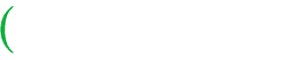Problem: Ms. Jensen likes to divide her class into groups of 2. Use mathematical symbols to represent all the students in her class.

Solution: Let g represent the number of groups in Ms. Jensen's class.

Then 2 · g, or 2g can represent "g groups of 2 students".

In the problem above, the variable g represents the number of groups in Ms. Jensen's class. A variable is a symbol used to represent a number in an expression or an equation. The value of this number can vary (change). Let's look at an example in which we use a variable.

Example 1: Write each phrase as a mathematical expression.

 Phrase Expression the sum of nine and eight 9 + 8 the sum of nine and a number x 9 + x

The expression 9 + 8 represents a single number (17). This expression is a numerical expression, (also called an arithmetic expression). The expression 9 + x represents a value that can change. If x is 2, then the expression 9 + x has a value of 11. If x is 6, then the expression has a value of 15. So 9 + x is an algebraic expression. In the next few examples, we will be working solely with algebraic expressions.

Example 2: Write each phrase as an algebraic expression.

 Phrase Expression nine increased by a number x 9 + x fourteen decreased by a number p 14 - p seven less than a number t t - 7 the product of 9 and a number n 9 · n   or   9n thirty-two divided by a number y 32 ÷ y   or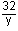In Example 2, each algebraic expression consisted of one number, one operation and one variable. Let's look at an example in which the expression consists of more than one number and/or operation.

Example 3: Write each phrase as an algebraic expression using the variable n.

 Phrase Expression five more than twice a number 2n + 5 the product of a number and 6 6n seven divided by twice a number 7 ÷ 2n   or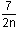three times a number decreased by 11 3n - 11Example 4: A small company has \$1000 to distribute to its employees as a bonus. How much money will each employee get?

Solution: Let e represent the number of employees in the company. The amount of money each employee will get is represented by the following algebraic expression: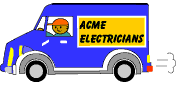Example 5: An electrician charges \$45 per hour and spends \$20 a day on gasoline. Write an algebraic expression to represent his earnings for one day.

Solution: Let x represent the number of hours the electrician works in one day. The electrician's earnings can be represented by the following algebraic expression:

Solution: 45x - 20

Summary: A variable is a symbol used to represent a number in an expression or an equation. The value of this number can change. An algebraic expression is a mathematical expression that consists of variables, numbers and operations. The value of this expression can change.

### Exercises

Directions: Choose the algebraic expression that correctly represents the phrase provided. Select your answer by clicking on its button. Feedback to your answer is provided in the RESULTS BOX. If you make a mistake, choose a different button.

 1. Fifteen less than twice a number 15 - 2x2x + 152x - 15None of the above. RESULTS BOX:
 2. Three times a number, increased by seventeen 3(a + 17)3a + 17(17 + 3)aNone of the above. RESULTS BOX:
 3. The product of nine and a number, decreased by six 9(m - 6)6(9 - m)9m - 6None of the above. RESULTS BOX:
4.  Thirty divided by seven times a number

 30 + 7n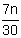30(7n) None of the above.
 RESULTS BOX:
5.  Jenny earns \$30 a day working part time at a supermarket. Write an algebraic expression to represent the amount of money she will earn in d days.

 30d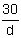30 + d None of the above
 RESULTS BOX: